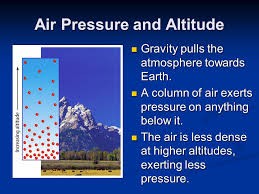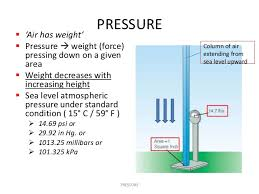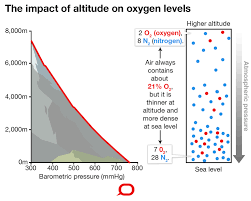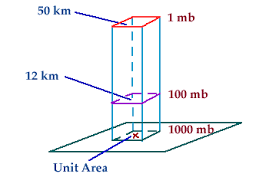# Air and the Adiabatic Effect.

29/12/2020

Did you know that Air has weight?  The most common definition of weight found in introductory physics textbooks defines weight as the force exerted on a body by gravity. This is often expressed in the formula W = mg, where W is the weight, m the mass of the object, and g gravitational acceleration.

The Weight of Air is "created" by the force of gravity (one of the four fundamental forces in physics). It "pulls" air molecules down towards its origin, from the outer reaches of the atmosphere to the actual surface where atmosphere meets the Earth. Because gravity diminishes with distance however, the "pull" at distances far away from the origin (ie. centre of Earth) is less than at the surface. This means air molecules can spread out more and exert less atmospheric pressure in doing so. It also means air exists in a column ranging from thin and voluminous down to thick and compressed.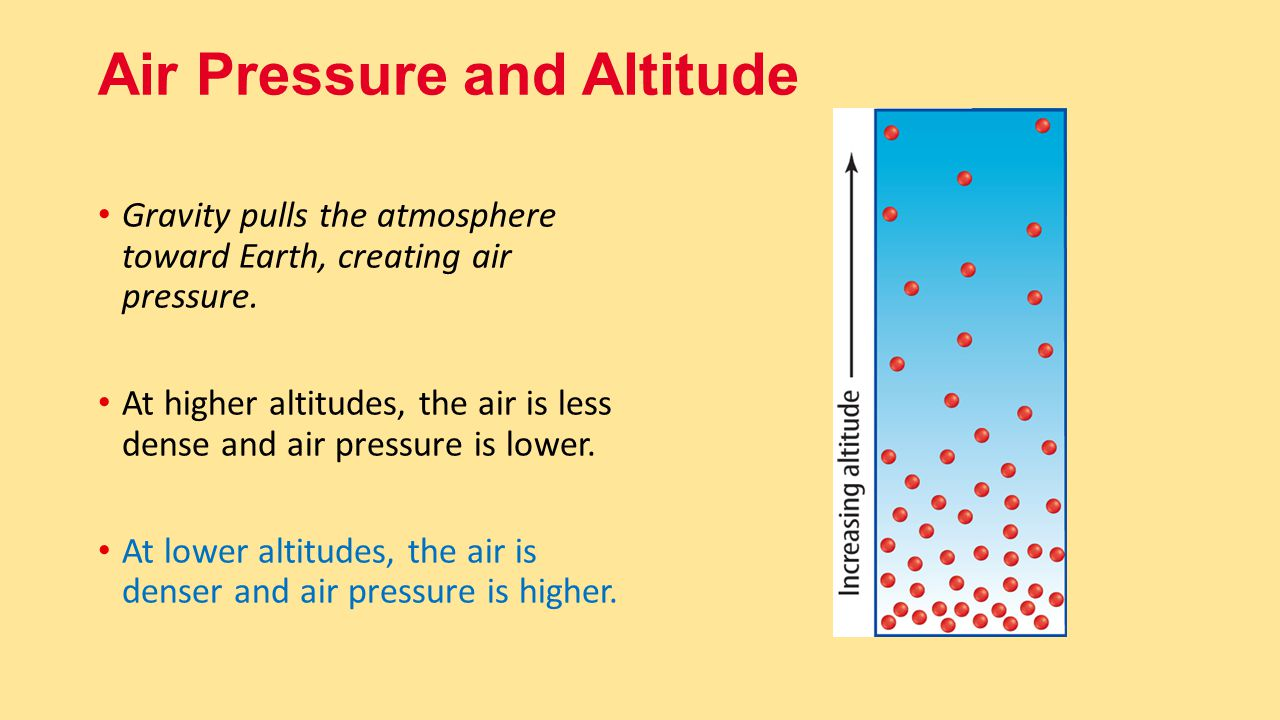At sea level, on average, the weight of the air above this unit area would weigh 14.7 pounds! The pressure applied by this air on the unit area would be 14.7 pounds per square inch. Meteorologists use a metric unit for pressure called a millibar. Average pressure at sea level is 1013.25 millibars. Note: 1 bar = 1 atm. = 14.7 # / in^2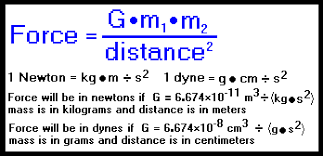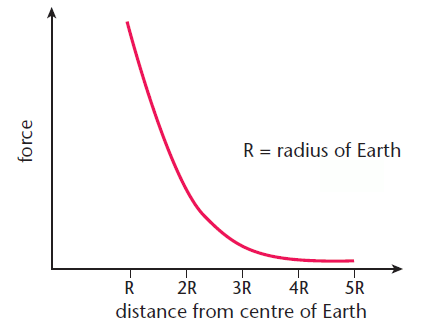In a column of air with an area of 1 square inch the pressure / weight of the air column stretching up to infinity is 14.7 lbs.  One mole of air (avagadro's number of molecules) is 30 grams / 454 = .064 lbs.  This means that a 1 inch square column of air with infinite height is composed of 229 moles of air stacked one on top of the other.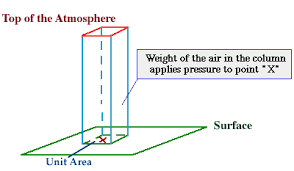Each mole occupies more volume than the one below it as pressure drops. Ie. avagadros number of molecules spread out as they occupy space higher up in the column. The density of a unit volume decreases.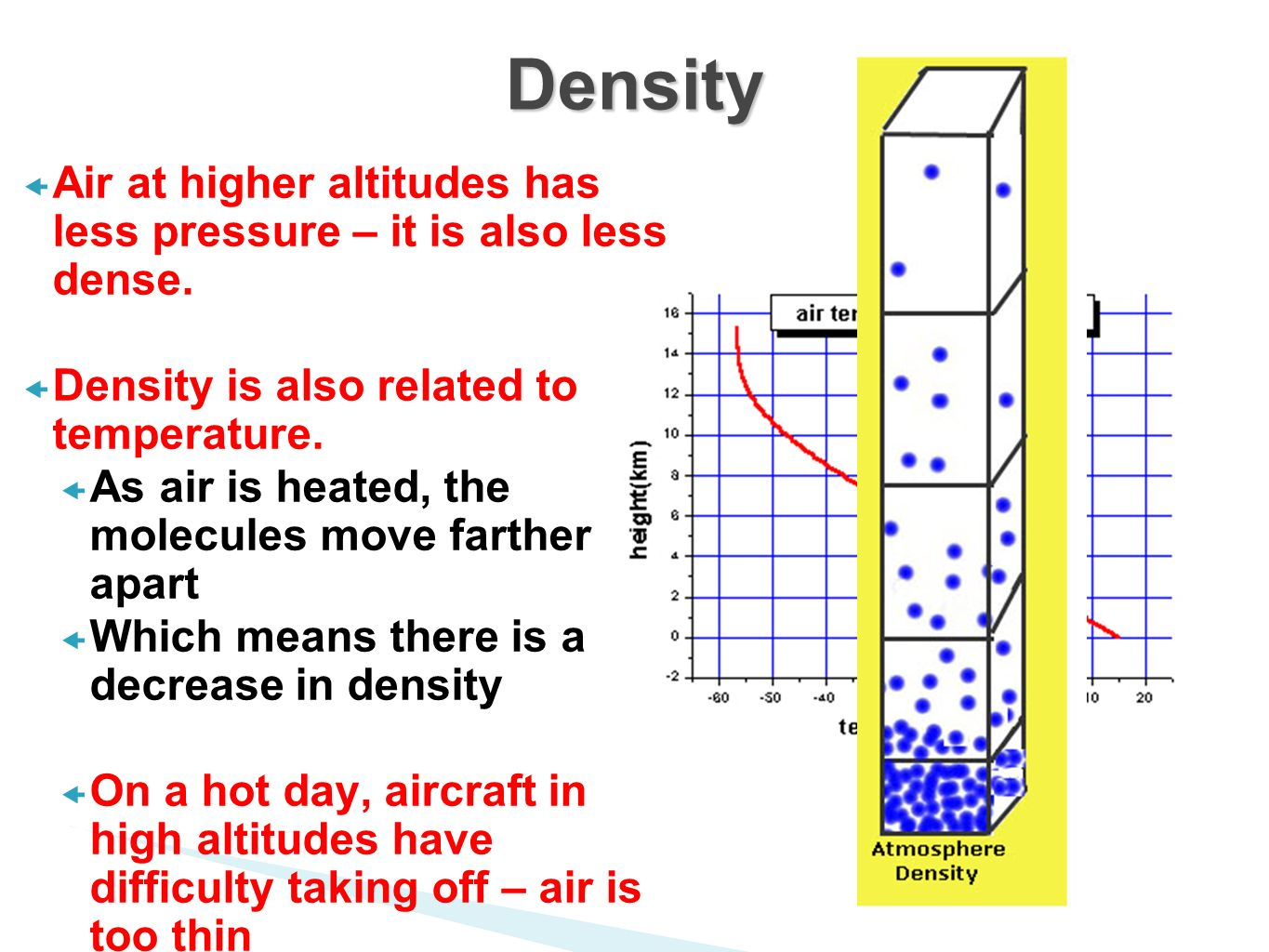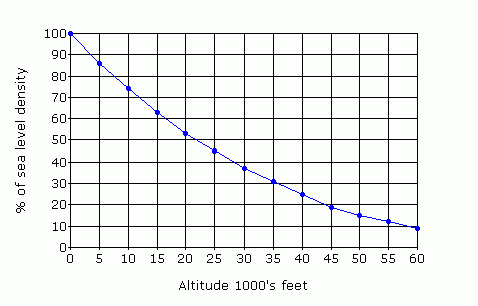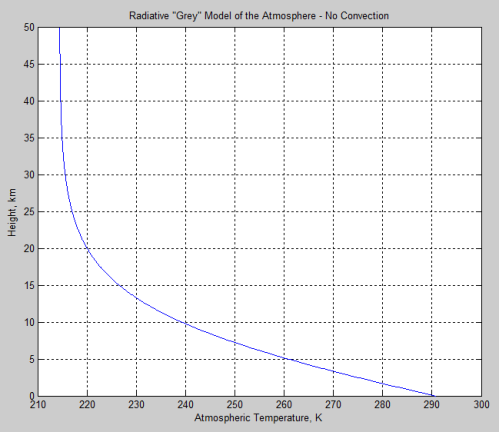Temperature is "created" by collisions of molecules with the tip of the thermometer. Collisions of molecules with the Thermometer bulb transfer energy to the fluid of the probe, which expands and gives a reading. The number of collisions is interpreted as a number we call temperature. The more the collisions the higher the number.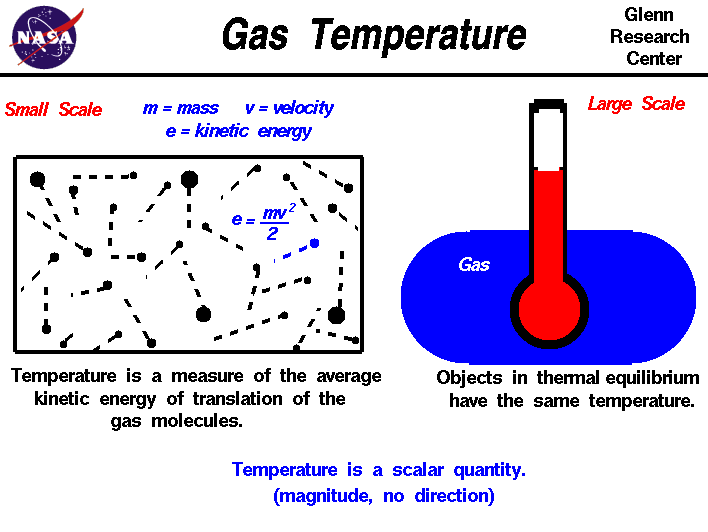Spreading out molecules (going up the column) means there is less chance of colliding with the thermometer. This means the number it will register will be lower.

Compressing molecules (bottom of the column) means that there is more chance of colliding with the thermometer. This means the number it will register will be higher.

Heating the column (without changing volume) will increase the rate at which molecules move and increase the chances of colliding with the thermometer tip. the number it registers will rise.

Heating the column (allowing the volume to increase) should (?) not change the number of collisions with the tip of the thermometer. the number should stay the same.  ???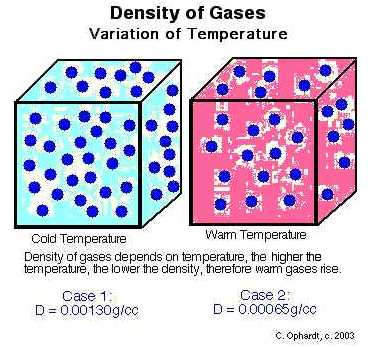Increasing the volume without increasing the avagadros number of molecules within it will decrease the number of collisions with the tip of the thermometer and the number it registers will decrease. (adiabatic cooling).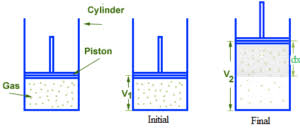RELEVANT DATA:

STP stands for Standard Temperature and Pressure. NTP stands for Normal Temperature and Pressure. STP is set by the IUPAC as 0°C and 100 kPa or 1 bar. NTPis set at 101.325 kPa but uses 20°C as the temperature.

28.9647 g/molThe molar mass of dry air with oxygen, nitrogen and the other components as indicated below is 28.9647 g/mol.

Avogadro's number, number of units in one mole of any substance (defined as its molecular weight in grams), equal to 6.02214076 × 1023. The units may be electrons, atoms, ions, or molecules, depending on the nature of the substance and the character of the reaction (if any). See alsoAvogadro's law.

Avogadro's number, number of units in one mole of any substance (defined as its molecular weight in grams), equal to 6.02214076 × 1023. The units may be electrons, atoms, ions, or molecules, depending on the nature of the substance and the character of the reaction (if any). See alsoAvogadro's law.

The physical principle known as Charles' law states that the volume of a gas equals a constant value multiplied by its temperature as measured on the Kelvin scale (zero Kelvin corresponds to -273.15 degrees Celsius).Nov 16, 1998

Boyle's Law states that the pressure (P) of a gas is inversely proportional to the volume (V). This law is valid as long as the temperature and the amount of gas are constant.

The gas laws consist of three primary laws: Charles' Law, Boyle's Law and Avogadro's Law (all of which will later combine into the General Gas Equation and Ideal Gas Law).Aug 21, 2020